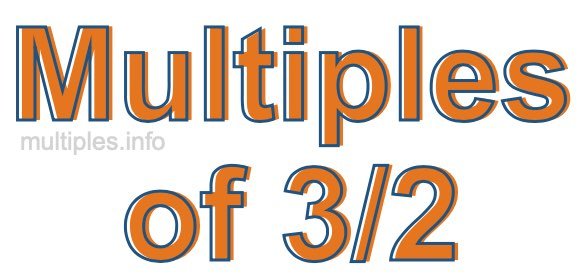Multiples of 3/2We define the multiples of 3/2 as the product of any integer multiplied by 3/2. Therefore, to create a list of multiples of 3/2 we start by multiplying 3/2 by one, then we multiply 3/2 by two, then we multiply 3/2 by three, and so on.

To multiply a fraction by an integer, we leave the denominator alone and multiply the numerator by the integer. It is impossible to give you a list of all multiples of 3/2 because the list goes on forever. However, here is the math to calculate the beginning list of multiples of 3/2.

3/2 × 1 = 3/2

3/2 × 2 = 6/2

3/2 × 4 = 9/2

3/2 × 4 = 12/2

3/2 × 5 = 15/2

3/2 × 6 = 18/2

3/2 × 7 = 21/2

3/2 × 8 = 24/2

3/2 × 9 = 27/2

3/2 × 10 = 30/2

3/2 × 11 = 33/2

3/2 × 12 = 36/2

We have simplified the fractions above for you. Below are the multiples of 3/2 in their lowest possible terms:

3/2 = 1 1/2

6/2 = 3

9/2 = 4 1/2

12/2 = 6

15/2 = 7 1/2

18/2 = 9

21/2 = 10 1/2

24/2 = 12

27/2 = 13 1/2

30/2 = 15

33/2 = 16 1/2

36/2 = 18

As we stated above, the list of multiples of 3/2 goes on forever because the product of 3/2 multiplied by any whole number (integer) is a multiple of 3/2. You can also create a list of multiples of 3/2 if you keep adding 3/2 like this:

3/2
3/2 + 3/2 = 6/2
3/2 + 3/2 + 3/2 = 9/2
3/2 + 3/2 + 3/2 + 3/2 = 12/2

Again, the list can go on forever, because you can continue adding 3/2 to the list. Therefore, the list of multiples of 3/2 is ∞ (infinite).

Multiples of Fractions
Do you need the multiples of another fraction? Enter a another fraction below to get the multiples of that fraction.

/

Multiples of 3/3
Go here for the next fraction on our list that we have multiples for.